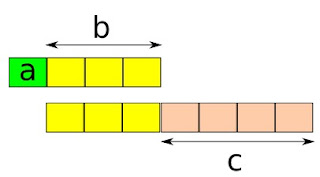### A simple ratio problem

Problem: If a:b = 1:3 and b:c = 3:4, find a:c.

Two ratios are given, third is to be found. This is very very simple. The picture shows the two given ratios as blocks.We can see that a is one block and c is four blocks, so the ratio a:c is 1:4.

You don't need an image for that, of course, since the original ratios are so easy. If a:b=1:3 and b:c=3:4, b being the same in both cases, we can write the ratio a:b:c as 1:3:4 right off.

But what if the numbers weren't so friendly? What if it said this way:

If a:b = 1:3 and b:c = 5:7, find a:c.

This is solvable in various ways. I'll use equivalent ratios, in other words change the given ratios to equivalent ratios until we find ones where the b's are the same.

In the first ratio, 1:3, b is 3. In the other ratio, 5:7, it is 5. We can make those to be 15 by changing the ratios to equivalent ratios - which is done in an identical manner as changing fractions to equivalent fractions.

1:3 = 5:15 and 5:7 = 15:21.

Now the ratio of a:b:c is 5:15:21, so the asked ratio a:c is 5:21.Anonymous said…
Hi Maria,

Thats a great way of solving ratio problems. One way I like to do these types of problems is by simply multiplying the two ratios. So in the above example, I know that if I multiply a/b and b/c, I will get a/c since b/b=1. Using this, I calculate 1/3 times 5/7, and get 5/21 which is the answer.Anonymous said…
Aren't equivalent ratios something like 1:2 and 2:4? And ratios are comparable to fractions so that 1:2 is like 1/2 and 2:4 is like 2/4.

I can't 'make' two fractions equivalent. I can test to see if they are equivalent though.

But what you did in your post worked. Isn't that more like finding the least common multiple between two numbers?

I haven't studied this in a while. I'm interested in your response!